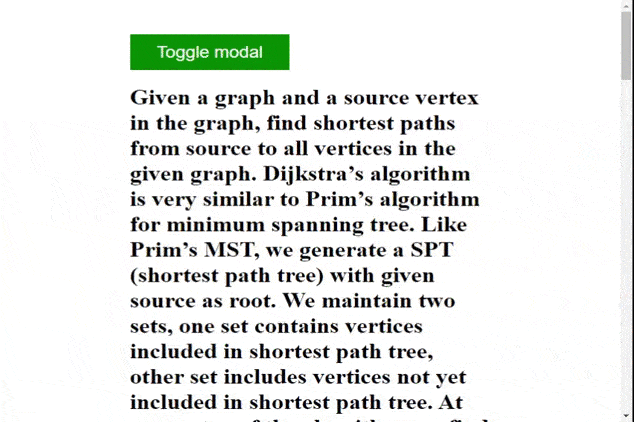Related Articles

# How to prevent Body from scrolling when a modal is opened using jQuery ?

• Last Updated : 12 Jan, 2021

Given an HTML document with a modal, the task is to prevent the body element from scrolling whenever the modal is in an open state. This task can be easily accomplished using JavaScript.

Approach: A simple solution to this problem is to set the value of the “overflow” property of the body element to “hidden” whenever the modal is opened, which disables the scroll on the selected element. Once the modal is closed, we would set the “overflow” property of the body element to “auto” so that scroll functionality is enabled on the body element. To find out whether the modal is opened or not, we would check if it has the “hidden” CSS class in its class list using the classList.contains() method of JavaScript. This “hidden” class is responsible for the opening and closing (changing display property) of the modal on a button click. Check out the given example for a better understanding.

Example:

## HTML

 ```<``html` `lang``=``"en"``>`` ` `<``head``>``    ``<``meta` `charset``=``"UTF-8"` `/>``    ``<``meta` `name``=``"viewport"` `content``=``        ``"width=device-width, initial-scale=1.0"` `/>`` ` `    ``<``style``>``        ``body {``            ``padding: 5% 20%;``        ``}`` ` `        ``#container {``            ``z-index: 1;``        ``}`` ` `        ``#btn {``            ``border: none;``            ``font-size: 24px;``            ``padding: 12px 36px;``            ``color: white;``            ``background-color: green;``        ``}`` ` `        ``#modal {``            ``height: 200px;``            ``width: 400px;``            ``padding: 60px;``            ``position: absolute;``            ``top: 50%;``            ``left: 50%;``            ``transform: translate(-50%, -50%);``            ``z-index: 999;``            ``background-color: green;``            ``color: white;``        ``}`` ` `        ``.hidden {``            ``display: none;``        ``}``    `````` ` `<``body``>`` ` `    ````    ``<``button` `id``=``"btn"` `onclick``=``"showModal()"``>``        ``Toggle modal``    ```` ` `    ````    ``<``div` `id``=``"modal"` `class``=``"hidden"``>``        ``<``div` `id``=``"modal-body"``>``            ``<``h1``>GeeksforGeeks``            ``<``h2``>This is a modal``        ````    ```` ` `    ````    ``<``div` `id``=``"container"``>``        ``<``h1``>``            ``Given a graph and a source vertex in ``            ``the graph, find shortest paths from ``            ``source to all vertices in the given ``            ``graph. Dijkstra’s algorithm is very ``            ``similar to Prim’s algorithm for minimum``            ``spanning tree. Like Prim’s MST, we ``            ``generate a SPT (shortest path tree) ``            ``with given source as root.``            ``We maintain two sets, one set contains ``            ``vertices included in shortest path tree,``            ``other set includes vertices not yet ``            ``included in shortest path tree. At every``            ``step of the algorithm, we find a vertex ``            ``which is in the other set (set of not yet``            ``included) and has a minimum distance from``            ``the source. Below are the detailed steps``            ``used in Dijkstra’s algorithm to find the``            ``shortest path from a single source vertex``            ``to all other vertices in the given graph.``            ``Algorithm Create a set sptSet (shortest ``            ``path tree set) that keeps track of vertices``            ``included in shortest path tree, i.e., whose``            ``minimum distance from source is calculated ``            ``and finalized. Initially, this set is empty.``            ``Assign a distance value to all vertices in ``            ``the input graph. Initialize all distance ``            ``values as INFINITE.``            ``Assign distance value as 0 for the source ``            ``vertex so that it is picked first. While ``            ``sptSet doesn’t include all vertices Pick a ``            ``vertex u which is not there in sptSet and ``            ``has minimum distance value. Include u to ``            ``sptSet. Update distance value of all adjacent ``            ``vertices of u. To update the distance values,``            ``iterate through all adjacent vertices. For ``            ``every adjacent vertex v, if sum of distance ``            ``value of u (from source) and weight of edge ``            ``u-v, is less than the distance value of v,``            ``then update the distance value of v.`` ` `            ``Given a graph and a source vertex in the ``            ``graph, find shortest paths from source to``            ``all vertices in the given graph. Dijkstra’s ``            ``algorithm is very similar to Prim’s ``            ``algorithm for minimum spanning tree.``            ``Like Prim’s MST, we generate a SPT (shortest ``            ``path tree) with given source as root.``            ``We maintain two sets, one set contains ``            ``vertices included in shortest path tree, ``            ``other set includes vertices not yet included``            ``in shortest path tree.``            ``At every step of the algorithm, we find a ``            ``vertex which is in the other set (set of not ``            ``yet included) and has a minimum distance ``            ``from the source.`` ` `            ``Below are the detailed steps used in ``            ``Dijkstra’s algorithm to find the shortest ``            ``path from a single source vertex to all other ``            ``vertices in the given graph. Algorithm``            ``Create a set sptSet (shortest path tree set) ``            ``that keeps track of vertices included in ``            ``shortest path tree, i.e., whose minimum ``            ``distance from source is calculated and ``            ``finalized. Initially, this set is empty. Assign ``            ``a distance value to all vertices in the input ``            ``graph. Initialize all distance values as INFINITE.``            ``Assign distance value as 0 for the source ``            ``vertex so that it is picked first. While sptSet ``            ``doesn’t include all vertices Pick a vertex u ``            ``which is not there in sptSet and has minimum ``            ``distance value. Include u to sptSet.``            ``Update distance value of all adjacent vertices ``            ``of u. To update the distance values, iterate ``            ``through all adjacent vertices. For every ``            ``adjacent vertex v, if sum of distance value ``            ``of u (from source) and weight of edge u-v, ``            ``is less than the distance value of v, then ``            ``update the distance value of v.``        ````    ```` ` `    ``<``script``>``         ` `        ``// Select required elements from the DOM``        ``const modal = document.querySelector("#modal");``        ``const body = document.querySelector("body");`` ` `        ``const showModal = function (e) {``            ``modal.classList.toggle("hidden");`` ` `            ``if (!modal.classList.contains("hidden")) {``                ``// Disable scroll``                ``body.style.overflow = "hidden";``            ``} else {``                ``// Enable scroll``                ``body.style.overflow = "auto";``            ``}``        ``};``    `````` ` ``

Output:Attention reader! Don’t stop learning now. Get hold of all the important HTML concepts with the Web Design for Beginners | HTML  course.

My Personal Notes arrow_drop_up